## Uniform polyhedra

It was proved in 1975 (by J. Skilling at the University of Cambridge) that, excluding the prisms and antyprisms, there are only 75 uniform polyhedra. A careful description of these was made earlier by Coxeter et al. (1954).

In the 1990's, I tried to build paper models of the sub-set that is mirror-symmetric (i.e., not including the objects without mirror symmetry, the snub polyhedra). I used mostly the nets in Magnus Wenninger's "Polyhedron Models" (Cambridge). And I almost finished the whole set! However, some of the more complex models proved to be too tedious, though, and I had other things to worry about. 3-D printing might be the way to do eventually finish this collection.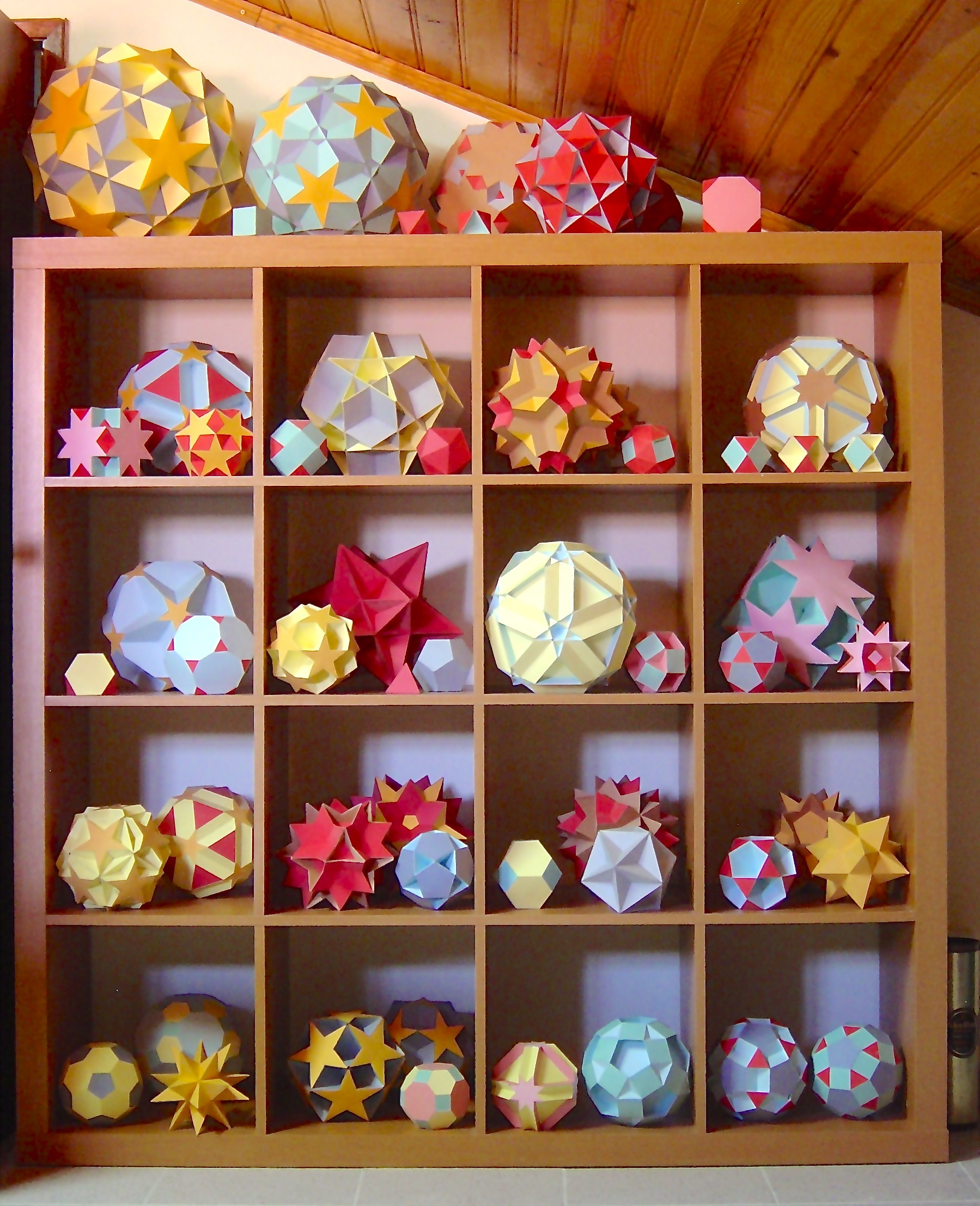Fig. 1: I made these 60 uniform polyhedra in the later 90's. These are in my parents' house in Portugal. This set includes the models of the regular and quasi-regular polyhedra displayed in my office, but on a smaller scale.

Another way of representing polyhedra is with edge models. One possibility is doing this with Zometool, another one, presented in the next figure, is 3-D printing. This model represents of two of the most complex uniform star polyhedra, which I have not built as paper models. It also represents two uniform polyhedron compounds.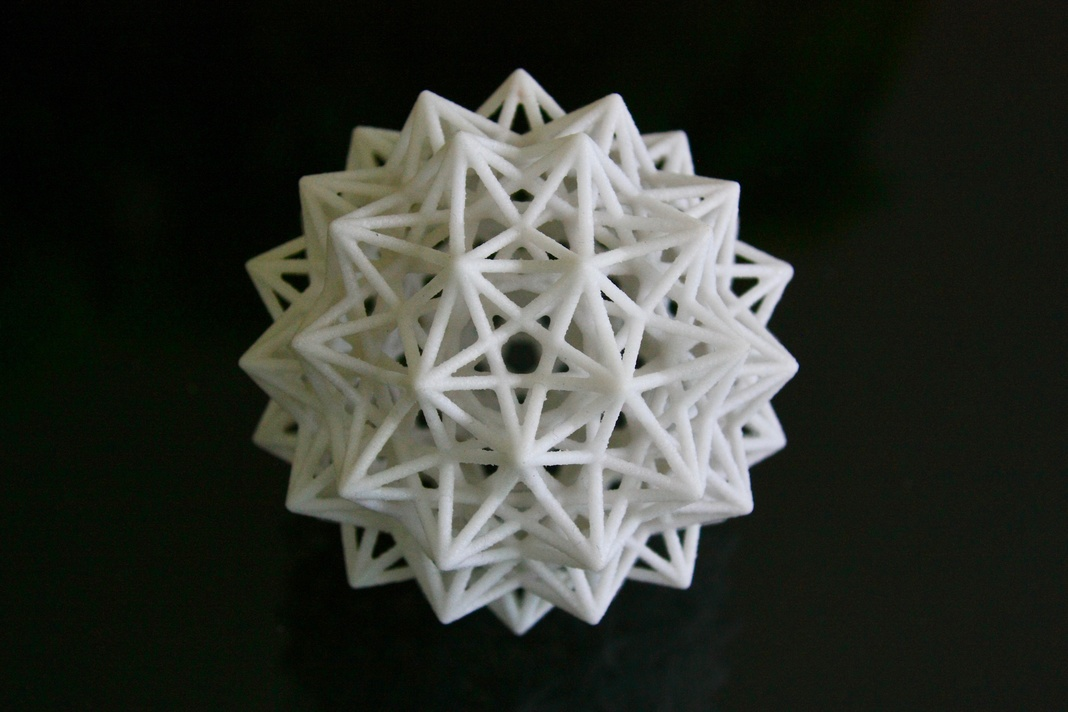Fig. 2: Here is a 3-D printed edge model of a complex uniform polyhedron, the Great dirhombicosidodecahedron, also known as "Miller's Monster". This was the last entry in the 1954 list of uniform polyhedra compiled by J. C. P. Miller, H. S. M. Coxeter and M. S. Longuet-Higgins.

The other remarkable polyhedron that shares this set of edges is the Great dis-nub dirhombidodecahedron. In 1975, John Skilling proved that the 1954 list of uniform polyhedra is complete. In that paper he generalized the concept of Uniform polyhedron: instead of two faces per edge, there can be any even number (or, equivalently, edges can be multiple). This relaxation yields only one additional polyhedron, the Great dis-nub dirhombidodecahedron.

Finally, the edges also belong to two different uniform polyhedron compounds: The Compound of twenty octahedra and the Compound of twenty tetrahemihexahedra.

Model printed by Shapeways, design by Mathematical Creations.

This video and the following pictures show how the paper models used to hang from the ceiling of the attic.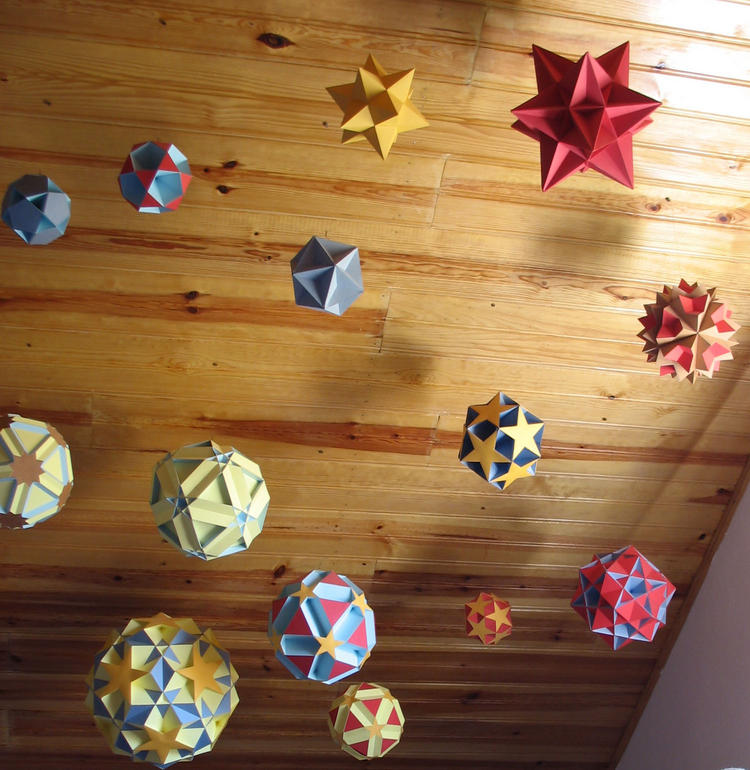In these pictures, all triangles are red, all squares are green, all pentagons are dark blue, all hexagons are yellow, all octagons are pink, all decagons are light blue, all pentagrams are gold, all octagrams are a lighter shade of pink and all decagrams are brown. These are the colors used in Roman Maeder's website. If a uniform polyhedron has a single type of face (i.e., a single color in this collection) then by definition it is regular.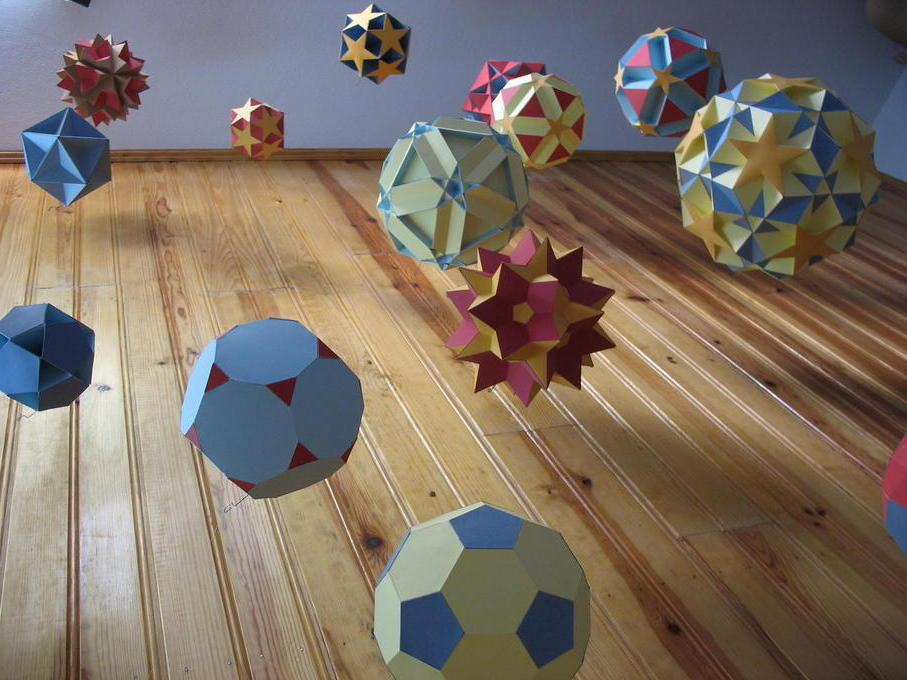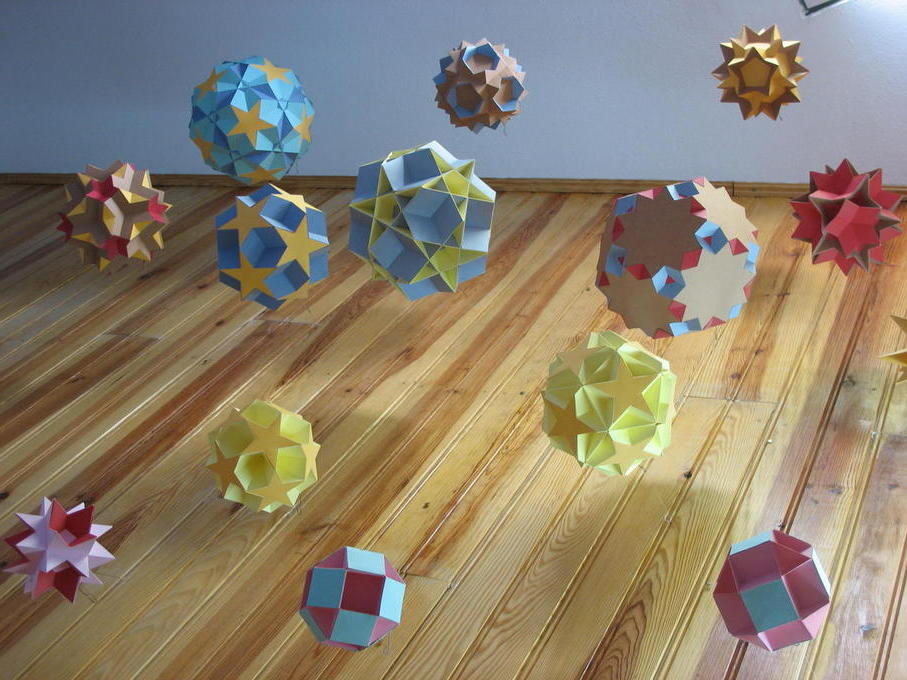Some of the complex, non-convex polyhedra with icosahedral symmetry.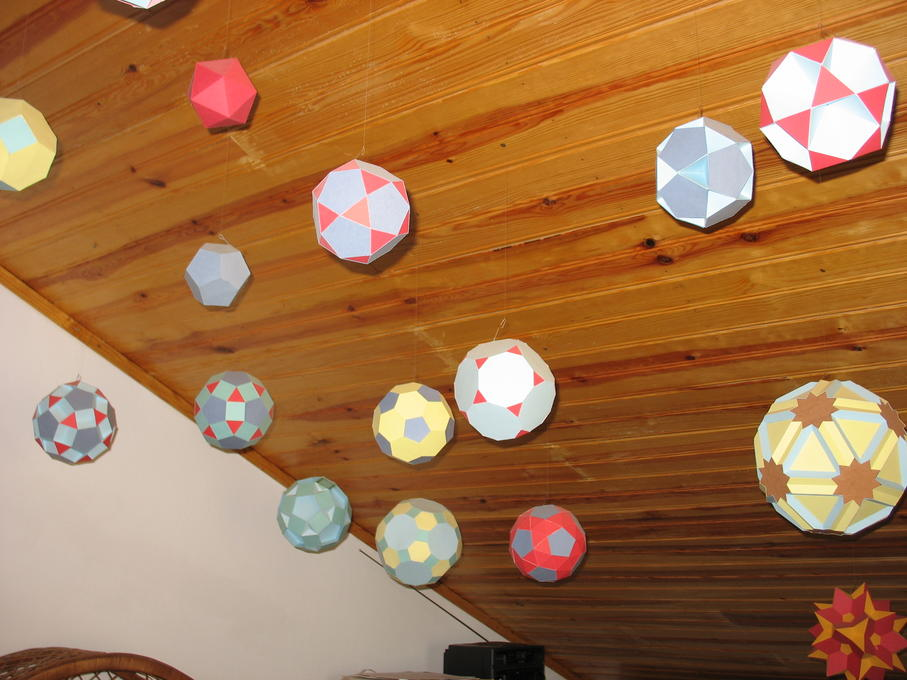The image above shows mainly Archimedean polyhedra with icosahedral symmetry. Among these are two regular polyhedra: the icosahedron (all in red) and the dodecahedron (all in dark blue).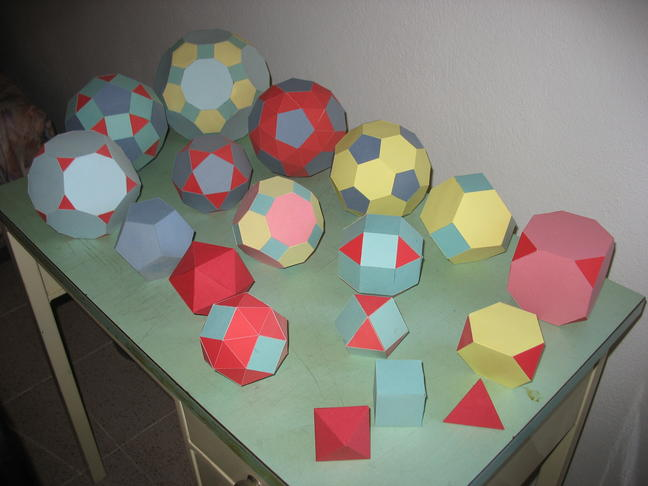The convex Uniform polyhedra: the five regular Platonic polyhedra and the 13 Uniform Archimedean polyhedra.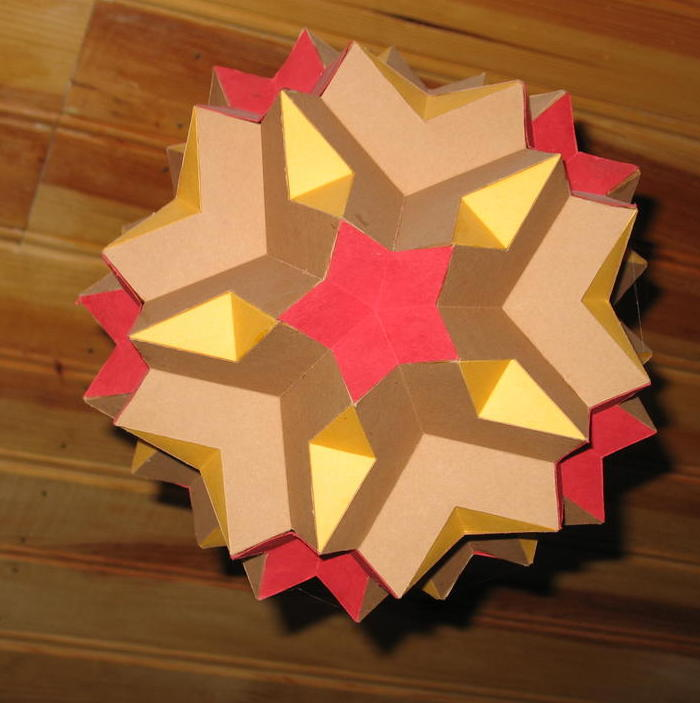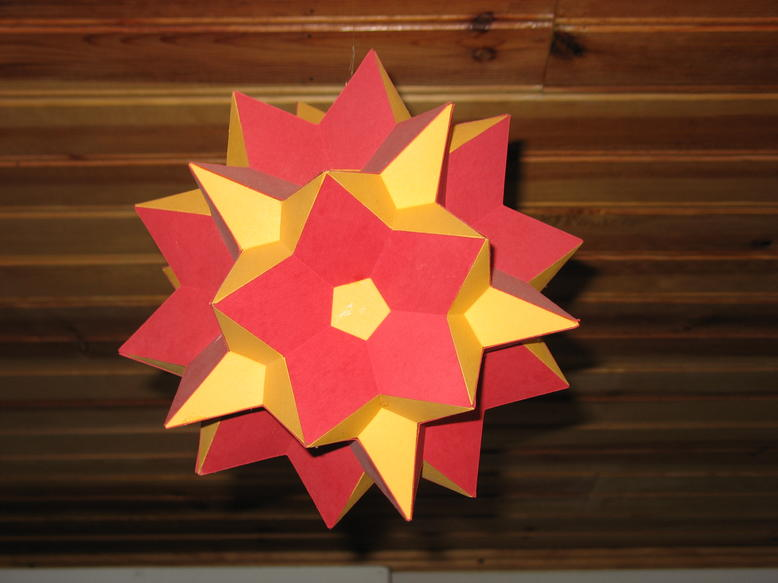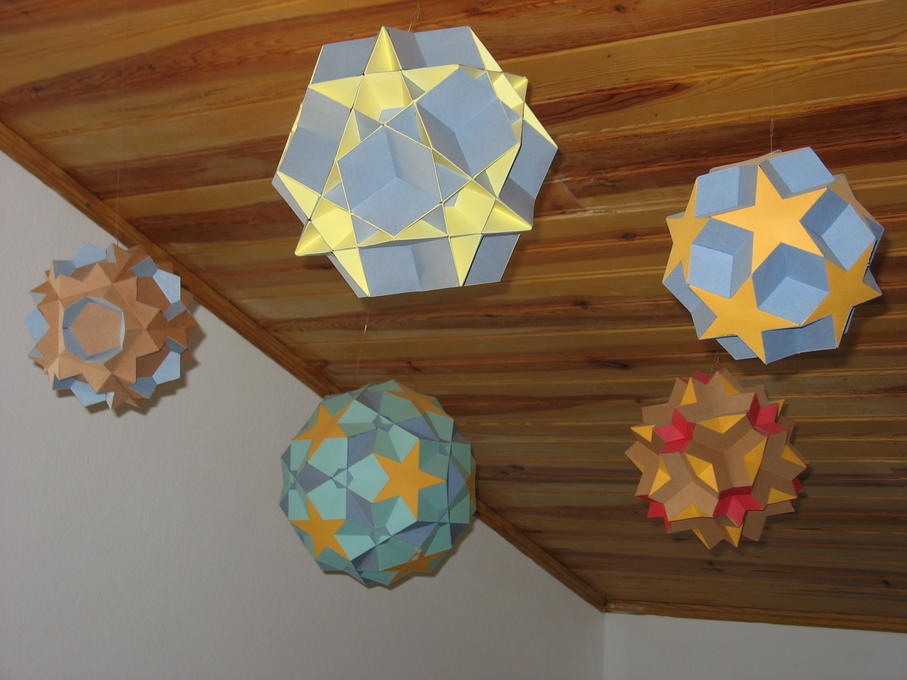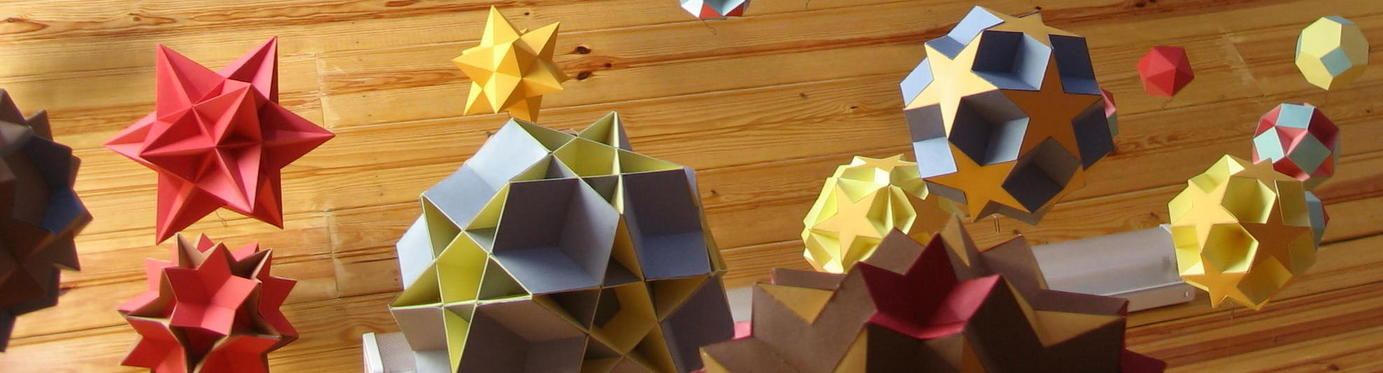A severe polyhedral storm!

Back to main polytope page.